# 5. Video Object Classification

## 5. Video Object Classification

The automatic segmentation procedure as described above provides object masks for each frame of the video. Based on these masks, further high-level processing steps are possible. To enable semantic scene analysis, it is required to assign object classes (e.g., persons, animals, cars) to the different masks. Furthermore, object behavior (e.g., a person is sitting, stands up, walks away) can be described by observing the object over time.

In our approach, object classification is based on comparing silhouettes of automatically segmented objects to prototypical objects stored in a database. Each real-world object is represented by a collection of two-dimensional projections (or object views). The silhouette of each projection is analyzed by the curvature scale space (CSS) technique. This technique provides a compact representation that is well suited for indexing and retrieval.

Object behavior is derived by observing the transitions between object classes over time and selecting the most probable transition sequence. Since frequent changes of the object class are unlikely, occasional false classifications resulting from errors which occurred in previous processing steps are removed.

### 5.1 Representing Silhouettes Using CSS

The curvature scale space technique [1, 13, 14] is based on the idea of curve evolution, i.e., basically the deformation of a curve over time. A CSS image provides a multi-scale representation of the curvature zero crossings of a closed planar contour.

Consider a closed planar curve Γ(u) representing an object view,

 (23.41)with the normalized arc length parameter u. The curve is smoothed by a one-dimensional Gaussian kernel g(u, σ) of width σ. The deformation of the closed planar curve is represented by

 (23.42)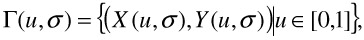where X(u,σ) and Y(u, σ) denote the components x(u) and y(u) after convolution with g(u,σ). Varying σ is equivalent to choosing a fixed σ' and applying the convolution iteratively.

The curvature k(u,σ) of an evolved curve can be computed using the derivatives Xu (u,σ), Xuu(u,σ), Yu(u,σ), and Yuu(u,σ) as

 (23.43)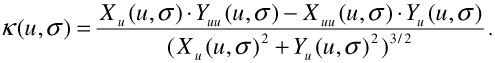A CSS image I(u,σ) is defined by

 (23.44)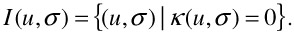It contains the zero crossings of the curvature with respect to their position on the contour and the width of the Gaussian kernel (or the number of iterations, see Figure 23.16). During the deformation process, zero crossings vanish as transitions between contour segments of different curvature are smoothed out. Consequently, after a certain number of iterations, inflection points disappear and the shape of the closed curve becomes convex. Note that due to the dependence on curvature zero crossings, convex object views cannot be distinguished by the CSS technique.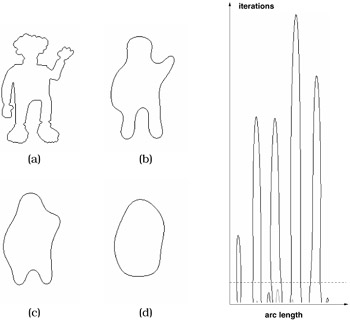Figure 23.16: Construction of the CSS image. Left— Object view (a) and iteratively smoothed contour (b)–(d). Right— Resulting CSS image.

Significant contour properties that stay intact for a large number of iterations result in high peaks in the CSS image. Segments with rapidly changing curvatures caused by noise produce only small local maxima. In many cases, the peaks in the CSS image provide a robust and compact representation of an object view's contour. Note that a rotation of an object view on the image plane can be accomplished by shifting the CSS image left or right in a horizontal direction. Furthermore, a mirrored object view can be represented by mirroring the CSS image.

Each peak in the CSS image is represented by three values, the position and height of the peak and the width at the bottom of the arc-shaped contour. The width specifies the normalized arc length distance of the two curvature zero crossings enframing the contour segment represented by the peak in the CSS image .

It is sufficient to extract the significant maxima (above a certain noise level) from the CSS image. For instance, in the example depicted in Figure 23.16, only five data triples remain and have to be stored after a small number of iterations. The database described in the following section stores up to 10 significant data triples for each silhouette.

### 5.2 Building the Database

The orientation of an object and the position of the camera have great impact on the silhouette of the object. Therefore, to enable reliable recognition, different views of an object have to be stored in the database.

Rigid objects can be represented by a small number of different views, e.g., for a car, the most relevant views are frontal views, side views, and views where frontal and side parts of the car are visible.

For non-rigid objects, more views have to be stored in the database. For instance, the contour of a walking person in a video changes significantly from frame to frame.

Similar views of one type of object are aggregated to one object class. Our database stores 275 silhouettes collected from a clip art library and from real-world videos. The largest number of images show people (124 images), animals (67 images), and cars (48 images). Based on behavior, the object class people is subdivided into the following object classes: standing, sitting, standing up, sitting down, walking, and turning around.

### 5.3 Object Matching

Each automatically segmented object view is compared to all object views in the database. In a first step, the aspect ratio, defined as quotient of object width and height, is calculated. For two object views with significantly different aspect ratios, no matching is carried out. If both aspect ratios are similar, the peaks in the CSS images of the two object views are compared. The basic steps of the matching procedure are summarized in the following :

• First, both CSS representations have to be aligned. For this purpose it might be necessary to rotate or mirror one of them. As mentioned above, shifting the CSS image corresponds to a rotation of the original object view. To align both representations, one of the CSS images is shifted so that the highest peak in both CSS images is at the same position.

• A matching peak is determined for each peak in a given CSS representation. Two peaks match if their height, position, and width are within a certain range.

• If a matching peak is found, the Euclidean distance of the peaks in the CSS image is calculated and added to a distance measure. If no matching peak can be determined, the height of the peak is multiplied by a penalty factor and added to the total difference.

### 5.4 Classifying Object Behavior

The matching technique described above calculates the best database match for each automatically segmented object view. Since the database entries are labeled with an appropriate class name, the video object can be classified accordingly. The object class assigned to a segmented object mask can change over time because of object deformations or matching errors. Since the probability of changing from one object class to another depends on the respective classes, we assign additionally matching costs for each class change.

Let dk(i) denote the CSS distance between an input object mask at frame i and object class k from the database. Furthermore, let wk,l denote the transition cost from class k to l (cf. Figure 23.17). Then, we seek the classification vector c, assigning an object class to each input object mask, which minimizesFigure 23.17: Weights for transitions between object classes. Thicker arrows represent more probable transitions.

 (23.45)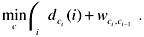This optimization problem can be solved by a shortest path search as depicted in Figure 23.18. With respect to the figure, dk(i) corresponds to costs assigned to the nodes and wk,l corresponds to costs at the edges. The optimal path can be computed efficiently using a dynamic programming algorithm. The object behavior can be extracted easily from the nodes along the minimum cost path.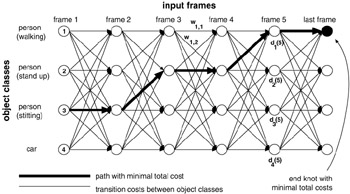Figure 23.18: Extraction of object behavior.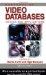Handbook of Video Databases: Design and Applications (Internet and Communications)
ISBN: 084937006X
EAN: 2147483647
Year: 2003
Pages: 393